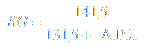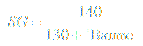# Specific gravity conversion calculator

## API and Baume specific gravity

### online since 2010## Calculator start selection

Read all about available deployments. In any way of utilizing calculator, Internet connection is not required, but nice to have for authentication at least.

#### When is this calculator suitable?

Specific gravity conversion calculator can be used for conversion between API degrees, Baume degrees and specific gravity and is applicable for all liquids, but most common application is for oils. Specific gravity is ratio of some liquid density divided by water density, at referenced temperature. As liquids are having different gravity on different temperature, for this calculator 60 degrees F (60 0F; 15.6 0C) is used as reference temperature - SG (60 0F/60 0F).

Calculator also calculates liquid density ρ in [lb/gallon] and specific volume v, in [barrels/MT] (barrels/metric tons) units.

Conversion in API degrees is for liquids with specific gravity lower than 1, which means for liquids lighter than water. Conversion in Baume degrees is for liquids with specific gravity higher or lower than 1, which means for liquids heavier or lighter than water.

#### Calculator description

Calculator use following equations for conversion between API and Baume degrees.

For oils specific gravity SG(60OF/60OF):For liquids lighter than water SG(60OF/60OF):For liquids heavier than water SG(60OF/60OF):As the density of water at 15OC is 999 kg/m3, density of oil or other liquid can be calculated as: water density x SG(60OF/60OF).

# Need a helping hand?If you need a quick calculation, but you are not still familiar how to use the calculator, you can order calculation service from the calculator developer.